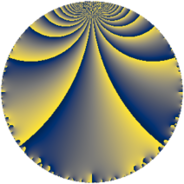Properties

 Label 1859.4.eLevel $1859$ Weight $4$ Character orbit 1859.e Rep. character $\chi_{1859}(529,\cdot)$ Character field $\Q(\zeta_{3})$ Dimension $772$ Sturm bound $728$

Related objects

Defining parameters

 Level: $$N$$ $$=$$ $$1859 = 11 \cdot 13^{2}$$ Weight: $$k$$ $$=$$ $$4$$ Character orbit: $$[\chi]$$ $$=$$ 1859.e (of order $$3$$ and degree $$2$$) Character conductor: $$\operatorname{cond}(\chi)$$ $$=$$ $$13$$ Character field: $$\Q(\zeta_{3})$$ Sturm bound: $$728$$

Dimensions

The following table gives the dimensions of various subspaces of $$M_{4}(1859, [\chi])$$.

Total New Old
Modular forms 1120 772 348
Cusp forms 1064 772 292
Eisenstein series 56 0 56

Trace form

 $$772 q + 4 q^{2} - 12 q^{3} - 1552 q^{4} + 16 q^{5} + 30 q^{6} - 68 q^{7} - 84 q^{8} - 3510 q^{9} + O(q^{10})$$ $$772 q + 4 q^{2} - 12 q^{3} - 1552 q^{4} + 16 q^{5} + 30 q^{6} - 68 q^{7} - 84 q^{8} - 3510 q^{9} + 4 q^{10} + 444 q^{12} + 120 q^{14} - 176 q^{15} - 6244 q^{16} + 44 q^{17} - 1136 q^{18} + 244 q^{19} - 124 q^{20} - 72 q^{21} - 44 q^{22} - 518 q^{23} + 240 q^{24} + 20300 q^{25} + 1704 q^{27} - 754 q^{28} - 180 q^{29} - 608 q^{30} - 576 q^{31} + 260 q^{32} - 72 q^{34} + 136 q^{35} - 15610 q^{36} + 1172 q^{37} + 2396 q^{38} - 1656 q^{40} - 272 q^{41} + 824 q^{42} + 644 q^{43} + 616 q^{44} - 240 q^{45} + 310 q^{46} - 812 q^{47} + 726 q^{48} - 18342 q^{49} - 1234 q^{50} - 3144 q^{51} + 376 q^{53} + 244 q^{54} - 440 q^{55} + 384 q^{56} - 1528 q^{57} - 1072 q^{58} + 1744 q^{59} + 748 q^{60} - 164 q^{61} + 72 q^{62} - 1540 q^{63} + 51916 q^{64} - 1144 q^{66} - 908 q^{67} + 866 q^{68} - 844 q^{69} + 1708 q^{70} - 274 q^{71} + 1190 q^{72} + 4168 q^{73} + 704 q^{74} + 1020 q^{75} + 320 q^{76} + 1232 q^{77} - 2832 q^{79} + 3902 q^{80} - 31090 q^{81} + 840 q^{82} - 1320 q^{83} + 780 q^{84} + 3168 q^{85} - 7856 q^{86} - 1576 q^{87} - 924 q^{88} + 2426 q^{89} - 23476 q^{90} - 4516 q^{92} - 3508 q^{93} + 3862 q^{94} - 3288 q^{95} + 1728 q^{96} + 746 q^{97} + 2086 q^{98} + O(q^{100})$$

Decomposition of $$S_{4}^{\mathrm{new}}(1859, [\chi])$$ into newform subspaces

The newforms in this space have not yet been added to the LMFDB.

Decomposition of $$S_{4}^{\mathrm{old}}(1859, [\chi])$$ into lower level spaces

$$S_{4}^{\mathrm{old}}(1859, [\chi]) \cong$$ $$S_{4}^{\mathrm{new}}(13, [\chi])$$$$^{\oplus 4}$$$$\oplus$$$$S_{4}^{\mathrm{new}}(143, [\chi])$$$$^{\oplus 2}$$$$\oplus$$$$S_{4}^{\mathrm{new}}(169, [\chi])$$$$^{\oplus 2}$$